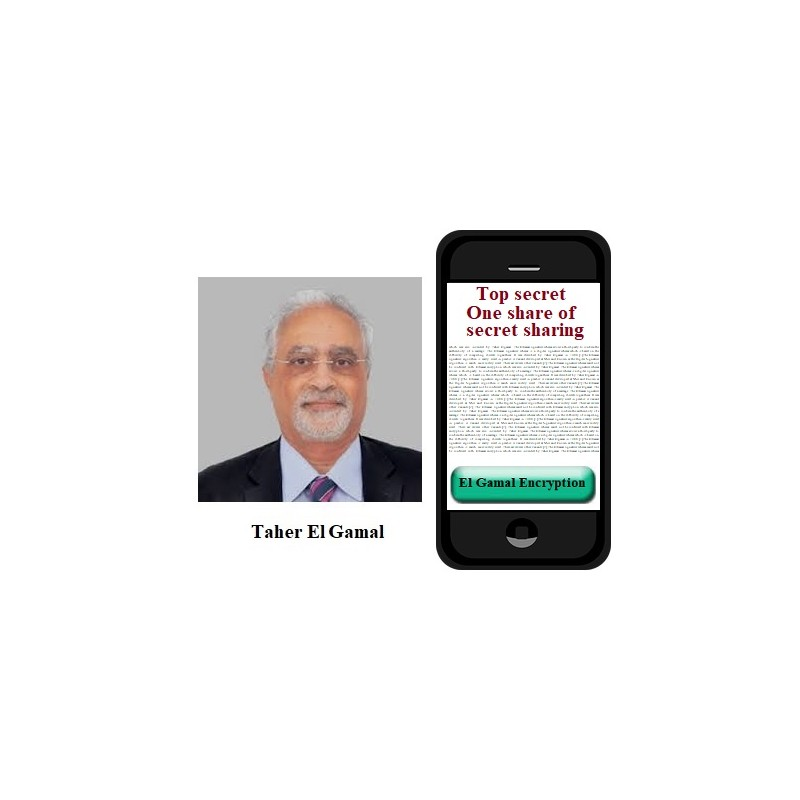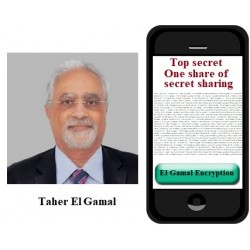•ElGamal encryption

€3.00
Tax included

ElGamal encryption scheme.

Quantity

COMPLETED.

This protocol is executed between Student Alice (You) and Mentor Bob. Parameter values sent by Alice are included in brackets [ ] and must be entered in the corresponding input fields.

The following functions are used in the protocol:

>> genstrongprime(bl)

>> mod_exp(g,x,p)

>> randi(z)

1. Generate strong prime number p of 28 bits length using Octave function

>> genstrongprime(28)

You could wait for a moment. You must check that generated p is greater than 2^27= 134217728. Send [p] to the Bob.

1. If it is OK, find a generator g of multiplicative group Zp={1,2,3, … p-1} and send system parameters [p, g] to the Mentor.

1. If it is OK, generate at random secret number x in the interval 1<x<p-1, compute Public Key A=gx mod p and send [A] to the Mentor.

1. Mentor sends to You his Public Key B=….. and ElGamal ciphertext C=(….., …..)=(C1, C2) of encrypted random number z. Decrypt this ciphertext and find z. Form a message DT consisting of current date and time in the format DT=MMDDhhmm . Compute message D=z+DT and Encrypt message D by computing ElGamal ciphertext CD=(CD1, CD2). Send [CD1, CD2] to the Mentor for verification.

3148 Items